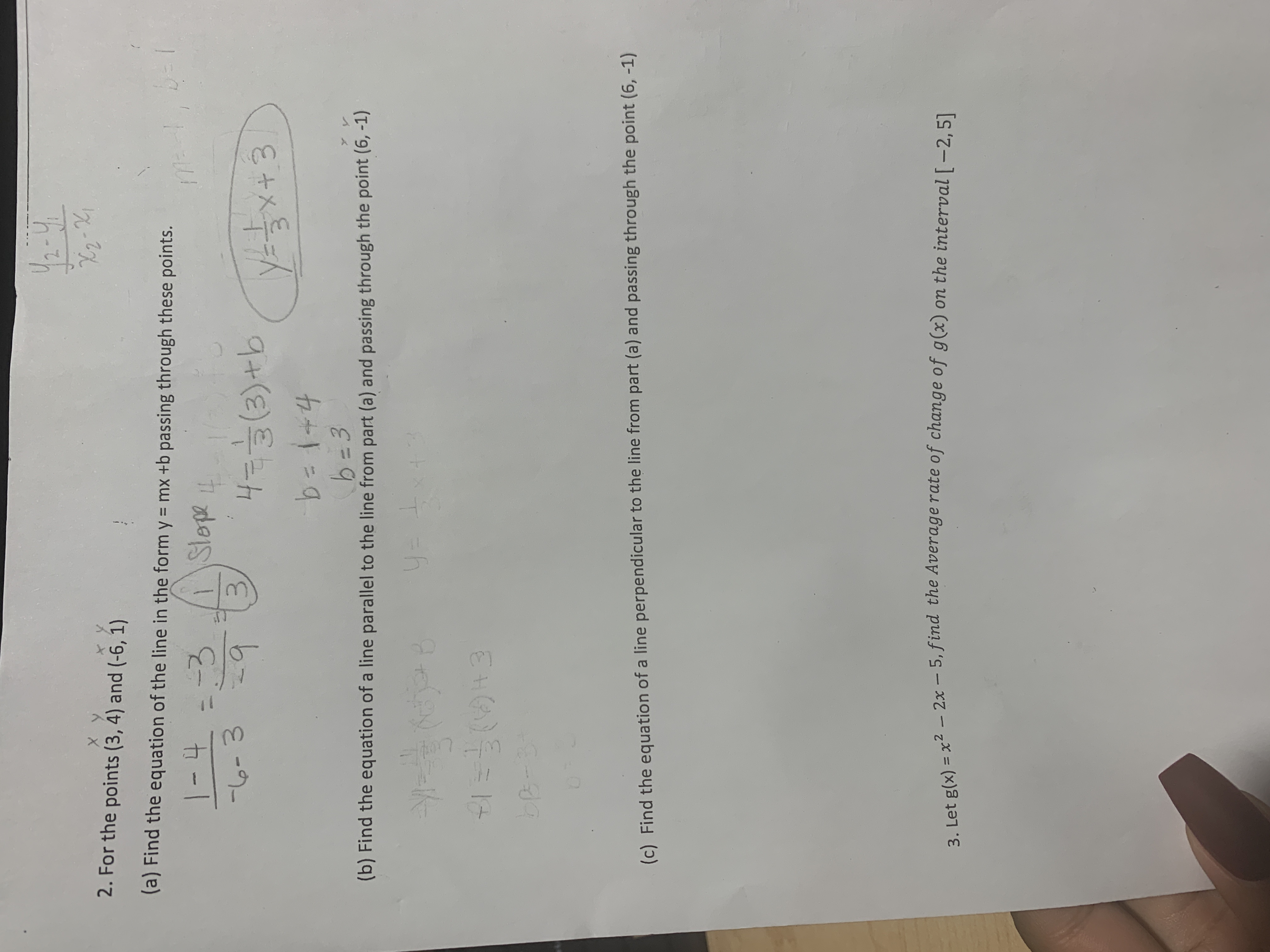# 2. For the points (3, 4) and (-6, 1)(a) Find the equation of the line in the form y = mx +b passing through these points.DE1-43-3.Slope30-34(3)tbbai+4b 3(b) Find the equation of a line parallel to the line from part (a) and passing through the point (6, -1)F1=0+3(c) Find the equation of a line perpendicular to the line from part (a) and passing through the point (6, -1)on the interval [ -2, 5]3. Let g(x) x - 2x - 5, find the Average rate of change of g(x)2

Question
6 views

I am having trouble with problems b, c, #3.help_outlineImage Transcriptionclose2. For the points (3, 4) and (-6, 1) (a) Find the equation of the line in the form y = mx +b passing through these points. DE 1-43 -3. Slope 3 0-3 4(3)tb bai+4 b 3 (b) Find the equation of a line parallel to the line from part (a) and passing through the point (6, -1) F1=0+3 (c) Find the equation of a line perpendicular to the line from part (a) and passing through the point (6, -1) on the interval [ -2, 5] 3. Let g(x) x - 2x - 5, find the Average rate of change of g(x) 2 fullscreen
check_circle

Step 1

The given points

(3, 4) and (-6, 1) passing through:

Step 2

Formula used:

Step 3

Put the value of the given points; calculat...

### Want to see the full answer?

See Solution

#### Want to see this answer and more?

Solutions are written by subject experts who are available 24/7. Questions are typically answered within 1 hour.*

See Solution
*Response times may vary by subject and question.
Tagged in

### Calculus